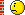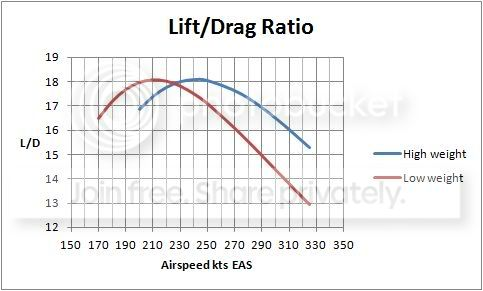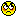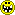> >>

# Why heavier aircrafts take longer to slow down in the air?

Tech Log The very best in practical technical discussion on the web

# Why heavier aircrafts take longer to slow down in the air?

Join Date: Jan 2008
Posts: 10,594
If that is true, would inertia in zero gravity space be equivalent to Vsquared ?

Inertia is zero gravity space is no different from inertia anywhere else. It's measured in kg. Nothing to do with V or V².Join Date: Jul 2009
Location: France - mostly
Age: 80
Posts: 1,689
Semantics

According to Wikipedia:
In common usage the term "inertia" may refer to an object's "amount of resistance to change in velocity" (which is quantified by its mass), or sometimes to its momentum, depending on the context. The term "inertia" is more properly understood as shorthand for "the principle of inertia" as described by Newton in his First Law of Motion; that an object not subject to any net external force moves at a constant velocity. Thus an object will continue moving at its current velocity until some force causes its speed or direction to change.Join Date: Aug 2007
Location: Derby UK
Age: 54
Posts: 112
inertia is mass and vice versa, momentum is mass or inertia x velocity

A moving body has momentum and kinetic energy, under the laws of conservation of energy, for the body to lose momentum, or velocity you have to move some of the kinetic energy into another form of energy.

Drag converts kinetic energy into other forms of energy such as heat, noise and kinetic energy in the air.

when the thrust equals the drag the body remains at a constant velocity.

Reduce thrust and the body will lose kinetic energy thru drag as heat etc, and will slow down until once again the thrust equals the drag. and we are again stable.

Now if you are in a glider the only thrust you have is in fact the momentum you have which is your mass x velocity in straight and level flight, or you can fall to earth and use G to give you acceleration which will again increase your velocity and hence your momentum. Or you can use a thermal say and raise your height increasing your potential energy which you can then exploit to convert it to kinetic energy once again, only to lose it as drag in the form of heat etc.

The key to understanding this is the conservation of energy and managing the energy available in whatever form you have it, it could be chemical energy in your fuel, or potential energy in your altitude or kinetic energy in your velocity and mass. BUT once you let it out of your system into another system in some form no use to you such as hot air or noise its very very hard to get it back to do work for you.

I'm not a pilot, i just design the hot bits that push you guys along, but i am pretty sure i'd rather have some altitude and speed in reserve as energy stores, just in case my bits let you down at the critical moment.

GBJoin Date: Dec 2010
Location: here and there
Age: 36
Posts: 34
Again, like HazleNut, assuming level flightLooking at the graph above...

Let's put a value of the minimum drag speed depicted on the diagram (courtesy of wikipedia) as 230kts for the heavier aircraft and 210kts for the lighter one.

So a speed of say 300kts will be a long way to the right of the minimum drag speed. As speed decreases AoA will increase. As AoA is increasing, initially its getting more to its optimum AoA. Form drag will be decreasing at a faster rate than induced drag is increasing ie. total drag is decreasing. As stated by Microburst the heavier aircraft, initially, is continuously flying closer to its optimum AoA than the lighter aircraft. Therefore it will be experiencing less total drag than the other aircraft and thus decelerating at a slower rate.

However once airspeed goes below Vmd for the heavier aircraft induced drag starts increasing at a faster rate than form drag is decreasing and so there is a rise in total drag. In the meantime however the lighter aircraft is now getting closer to its Vmd and so this aircraft will now start decelerating at a slower rate. Once both aircraft are below Vmd, I could well be wrong here though, I guess induced drag becomes the major player, the heavier the weight the more lift required. More lift = more induced drag = an increased rate of deceleration for the heavier aircraft.Join Date: Aug 2012
Location: Overland Park, KS
Posts: 120
Gee...People, are you serious to discuss this subject for 3 pages?
P = mv, where P - momentum, m - inertial mass, v - speed.

1.
Putting aside drag and all aerodynamics, and using the formula above we have:
The faster you go the more momentum you gain, OR
More mass you have, more momentum you obtain when moving with the same speed.

2. If we also have aerodynamics involved here, then we should separately do part 1, and then figure out drag. After that we can decide whether bigger AC can slow down faster than smaller one or not.

But drag doesn't change P given constant V and m.

Is not it that obvious?Last edited by Sunamer; 23rd Nov 2012 at 01:55.Join Date: Mar 2005
Location: Uh... Where was I?
Posts: 1,342
If I had my books and my scanner, I would post here the L/D curve, and a graph of an airplane gliding angle.

If an airplane has a Lift of 10 tons, and a drag of 1 ton, it has a L/D of 10 and it glides at an gradient of 10%. That is a fact. It takes some graphics and formulas, though.

The same airplane weighing 20 tons and with a drag of 2 tons, has the same L/D ratio, and therefore glides exactly the same, at a 10% gradient.

What's more. A 1 ton cessna with a 100 kg drag will have the same L/D ratio of 10 and glide at 10% gradient.

L/D ratio determines glide angle.

The L/D ratio is equivalent to the CL/CD ratio. CL and CD only depend on AoA, therefore L/D only depends on AoA, for a given configuration. Not on weight, not on speed. Only on AoA.

For 1g flight at a given weight, there is a relationship between speed and AoA, so that to each speed corresponds an AoA. Therefore, to each speed during a 1g glide at a given weight corresponds a L/D ratio.

Normally airplanes fly at AoAs smaller than max L/D AoA. When flying at AoAs higher than that, we are said to be in the "reverse command region", or the "back side of the curve". So an increase in speed is always decreasing the AoA and the L/D ratio, and therefore increases the glide angle (reduces the gliding distance).

The AoA-Speed relationship at 1g flight varies with weight. At increased weights, increased AoA are necessary to increase Lift in the same amount for a constant speed.

If you glide at the same speed but higher weight, your AoA is higher, and your L/D ratio increases, and so your glide angle reduces (gliding distance increases).

Now think of two big football balls (football balls are spheric, ok? I don't know nor I want to know what "soccer" is...) One filled with lead, the other with air. Which one will travel faster on a given slope? And Which one will need less slope to maintain a given speed? Well, the heavier needs a shallower slope because its weight can overcome more drag than the heavy one, right?.

Now, from the energy point of view you might have trouble understanding, because here there is a lot of energy dissipation. Actually, descent is all about energy dissipation (you glide at quite a constant speed and yet lose altitude, right?). You can't use energy conservation here...

finally, two airplanes of same model flying at maximum glide speed (min drag speed or max L/D speed) will glide the same, irrespective of the weight. Only the heavier will fly faster.

Last edited by Microburst2002; 23rd Nov 2012 at 06:39.Join Date: Jul 2009
Location: France - mostly
Age: 80
Posts: 1,689
yippy ki yay,

If I understand you correctly, I think you agree with Microburst and me. Just a small correction:
As stated by Microburst the heavier aircraft, initially, is continuously flying closer to its optimum AoA than the lighter aircraft. Therefore it will be experiencing less total drag than the other aircraft and thus decelerating at a slower rate.
Actually, above the minimum drag speed the heavier aircraft will have more total drag than the lighter one, but its drag-to-weight ratio will be lower and therefore it decelerates at a slower rate.

Sunamer,

1. Agreed
2. That's what we do

The reason for discussing it for so long is that it is not generally true to say that the heavier aircraft decelerates more slowly than the lighter one.

Microburst2002,
Normally airplanes fly at AoAs smaller than max L/D AoA.
True, for transport aircraft, except in a few minutes after takeoff and prior to landing. Gliders fly below minimum drag speed when circling in thermals to gain height.

Regards,
HN

Last edited by HazelNuts39; 23rd Nov 2012 at 07:26.Join Date: Jun 2011
Location: West of Offa's dyke
Age: 83
Posts: 474
Microburst

If I had my books and my scanner, I would post here the L/D curve, and a graph of an airplane gliding angle.

finally, two airplanes of same model flying at maximum glide speed (min drag speed or max L/D speed) will glide the same, irrespective of the weight. Only the heavier will fly faster.
This what you want?At anything above 230 kts on this aircraft the Lift/Drag ratio will be better at the higher weight and the aircraft will take longer to slow down;below that speed the opposite is true.

Last edited by Owain Glyndwr; 23rd Nov 2012 at 08:12.Join Date: Jan 2008
Posts: 10,594
Now if you are in a glider the only thrust you have is in fact the momentum you have
You were doing OK, up until that partJoin Date: Jan 2008
Location: Boston
Posts: 31
I've seen the name Newton come up. Where is Bernoulli? Also, where does the conveyor belt come into this?Join Date: Mar 2005
Location: Uh... Where was I?
Posts: 1,342
Bernoulli has absolutely nothing to do here. Like I said, gliding at constant speed is about energy dissipation. Bernouilli is a particular case of the energy conservation theorem, so they are incompatible things...

As for the conveyor belt, I have no idea! what is that?Join Date: Oct 2007
Location: Dubye
Posts: 94
The formula for drag is: Cd=1/2Rho x V(sq) x S

Rho = Air density
V= velocity
S= area

By increasing your speed, you increase your drag exponentially and therefore increase your rate of descent.Join Date: Nov 2012
Location: awaiting position fix
Posts: 8
I don't mean to be fussy, but Cd = Measured drag / Theoretical drag
Drag = Cd x 1/2 rho x V squared x S

Higher weight means a higher wing load allowing a further glide distance / time to slow down?Join Date: Aug 2012
Location: Overland Park, KS
Posts: 120
To HazelNuts39,
The reason for discussing it for so long is that it is not generally true to say that the heavier aircraft decelerates more slowly than the lighter one.
Agreed. When considering AoA of empty AC, AoA may be less, thus producing more efficient aerodynamic profile, than when the same AC weighs more, so it needs more AoA to compensate for more mass = more lift is needed.
That's my understanding.Join Date: Jul 2009
Location: France - mostly
Age: 80
Posts: 1,689
Originally Posted by Sunamer
When considering AoA of empty AC, AoA may be less, thus producing more efficient aerodynamic profile, than when the same AC weighs more, so it needs more AoA to compensate for more mass = more lift is needed.
That is true at any speed. For the variation of L/D in Owain Glyndwr's graph the wing's aspect ratio (roughly the ratio between wing span and average chord length) determines the efficiency of producing lift. As such it is perhaps as important as the 'efficient aerodynamic profile'.

Regards,
HN

Last edited by HazelNuts39; 23rd Nov 2012 at 16:09.Join Date: Aug 2007
Location: Derby UK
Age: 54
Posts: 112
Now if you are in a glider the only thrust you have is in fact the momentum you have
You were doing OK, up until that part
Please explain why that is wrong Dave.

GBJoin Date: Jan 2008
Posts: 10,594
Thrust and momentum are completely different quantities, with different units/dimensions.

A glider usually has momentum, but has no thrust (unless it has a pop-up sustainer or SL engine).

Good illustration here

L/D Ratio

of the three forces (lift, drag, weight) acting on a glider.Join Date: Aug 2007
Location: Derby UK
Age: 54
Posts: 112
We have 2 aircraft
plane A weighs 300 Tonnes and plane B weighs 200 Tonnes.
Both are identical and have the same wing with the same Lift drag ratio of 17 similar to a 747.
Both aircraft are cruising straight and level at 400 Km/h.
Plane A at 300 tonnes lift has a drag of 300 / 17 which equals 17.65 units of drag.
Plane B at 200 tonnes lift has a drag of 200 / 17 which equals 11.76 units of drag.
So plane A has 1.5 times more drag than Plane B. at this speed.
Plane A has a momentum of Mass x Velocity which is 300 x 400 = 120,000.
Plane B has a momentum of 200 x 400 = 80,000
So again we have plane A with 1.5 times the momentum of plane B.
So all is nicely in equilibrium with both planes nicely in straight and level flight.
Now we want to reduce the speed of both aircraft to 300 kmh.
Plane A has a kinetic energy of ½ x 300 x (400 x 400) = 24,000,000
At 300 kmh it will have KE of ½ x 300 x (300 x 300) = 13,500,000.
This means plane A has to lose 10,500,000 units of KE.
Plane B has a KE of ½ x 200 x (400 x 400) = 16,000,000
At 300 Kmh it will be ½ x 200 x (300 x 300) = 9,000,000
So plane B has to lose 7,000,000 units of KE.
Plane A has to lose 1.5 times the energy as plane B.
So as long as the lift drag ratio stays the same between the 2 velocities and the amount of lift needed, both aircraft will slow down at exactly the same rate.
If the lift drag ratio alters due to plane configuration it will affect the rate of deceleration between the 2 aircraft.
BUT maximum lift drag ratio is independent of the weight of the aircraft, the area of the wing, or the wing loading.

ive tried to keep this simple so forgive the rubbish maths, but I thought Id give you all something to pull apart for a while.
GBJoin Date: Aug 2007
Location: Derby UK
Age: 54
Posts: 112
Thrust and momentum are completely different quantities, with different units/dimensions.

A glider usually has momentum, but has no thrust (unless it has a pop-up sustainer or SL engine).

Good illustration here

L/D Ratio

of the three forces (lift, drag, weight) acting on a glider.
Sorry Dave but you're wrong here.

There are 4 forces acting on a gliding some of the time.

Your nasa illustration shows a gliding falling from the sky, BUT if he builds up some speed ( momentum ) and pulls back on the stick good n hard what happens, well he will go back up again against gravity untill he runs out of velocity at Agogee and then gravity will take over again and he will fall to earth again

During this upwards flight bit he is using the momentum built up as THRUST, and yes its called thrust becuase the momentum is PUSHING the glider upwards against gravity with the kinetic energy being converted to potential energy that is known as height, as is the tension on a kite string that stops it fluttering away in the wind.

so during this upwards flight mode using the momentum as thrust 4 forces are acting on the glider, not 3.

GBJoin Date: Jun 2011
Location: West of Offa's dyke
Age: 83
Posts: 474
@Bye
Thrust and momentum are completely different quantities, with different units/dimensions.Sorry Dave but you're wrong here.
Dave is right and you are wrong I'm afraid.

Thrust (like any force) is a rate of change of momentum not momentum. Different units and different dimensions.

We have 2 aircraft
plane A weighs 300 Tonnes and plane B weighs 200 Tonnes.
Both are identical and have the same wing with the same Lift drag ratio of 17 similar to a 747.
Both aircraft are cruising straight and level at 400 Km/h.
Plane A at 300 tonnes lift has a drag of 300 / 17 which equals 17.65 units of drag.
Plane B at 200 tonnes lift has a drag of 200 / 17 which equals 11.76 units of drag.
Wrong again. The 300 tonne aircraft will have a lift coefficient 1.5 times greater than the 200 tonne if they are flying at the same speed. They cannot have the same drag coefficient or the same lift/drag ratio so the rest of the argument falls by the wayside - even without perpetuating the thrust = momentum error.

And just for the record, the 'heavy' aircraft in the picture I posted earlier was 1.3 times the weight of the 'light' one

Last edited by Owain Glyndwr; 23rd Nov 2012 at 18:46.Show Printable VersionEmail this Page